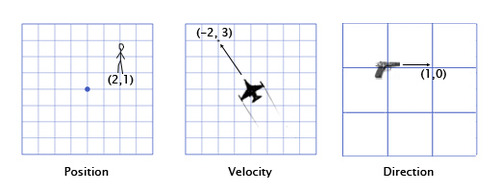Linear algebra has a significant role in game development; it encompasses relevant math concepts such as linear sets of equations and their transformation properties. Linear algebra allows the analysis of rotations in space, least squares fitting, solution of coupled differential equations, determination of a circle passing through three given points, as well as many other problems in mathematics, physics, and engineering. Simply put, linear algebra is the mathematics branch concerning vector spaces and the linear mappings between them.

These articles aim to create the foundation for 2D & 3D game development. Therefore, rather than just talking about graphic API functions and how to use them, let us take a deeper look to the math used under the hood.

### What is a vector?

Vectors are the formal mathematical entities we use to do 2D and 3D math. The word vector has two distinct, but related, meanings. Mathematically, a vector is a set of numbers. Context gives relevance to these numbers; for example, in a given context a vector could represent a position, a direction, or a velocity.Image Source: Wolfire Games

Geometrically, a vector is a directed line segment that has a magnitude and a direction.

• The magnitude of a vector is the length of the vector. A vector may have any non-negative length.

• The direction of a vector describes which way the vector is pointing in space. Note that direction is not exactly the same as orientation.

#### Vector vs. Scalar

A scalar is a quantity that is invariant under rotations of the coordinate system. We use this term specifically when we wish to emphasize that a particular quantity is not a vector quantity. For example, "velocity" and "displacement" are vector quantities, while "speed" and "distance" are scalar quantities.

#### Dimension

The dimension of a vector tells how many numbers or components the vector contains. Vectors may be of any positive dimension, including one. In fact, a scalar is a one-dimension vector.

#### Vectors vs. Points

Vectors describe displacements, and therefore, they can describe relative positions. (Walk 3 steps [magnitude] forward [direction]) Points specify positions. Points, like vectors, give relative positions. Points are relative to the origin of the coordinate system used to specify their coordinates.### What is a matrix?

In linear algebra, a matrix is a rectangular grid of numbers arranged into rows and columns. You could say that a vector is an array of scalars, and a matrix is an array of vectors. A square matrix is a matrix with the same amount of rows and columns.

#### Linear Transformations

In general, a square matrix can describe any linear transformation. Linear transformations preserve straight and parallel lines, and there is no translation. The origin does not move. While a linear transformation preserves straight lines, other properties of the geometry, such as lengths, angles, areas, and volumes, are possibly altered by transformation. In a non-technical sense, a linear transformation may "stretch" the coordinate space, but it does not curve or warp it.

#### Affine Transformations

An affine transformation is any transformation that preserves collinearity (i.e., all points lying on a line initially still lie on a line after transformation) and ratios of distances (e.g., the midpoint of a line segment remains the midpoint after transformation). In this sense, affine indicates a special class of projective transformations that do not move any objects from the affine space Rᶟ to the plane at infinity or conversely. An affine transformation is also called an affinity.

Geometric contraction, expansion, dilation, reflection, rotation, shear, similarity transformations, spiral similarities, and translation are all affine transformations, as are their combinations. In general, an affine transformation is a composition of rotations, translations, dilations, and shears.

#### Things to keep in mind

• The rows of a square matrix can be interpreted as the basis vectors of a coordinate space.
• To transform a vector from the original coordinate space to the new coordinate space, we multiply the vector by the matrix.
• The transformation from the original coordinate space to the coordinate space defined by these basis vectors is a linear transformation. A linear transformation preserves straight lines, and parallel lines remain parallel. However, angles, lengths, areas, and volumes may be altered after transformation.
• Multiplying the zero-vector by any square matrix results in the zero-vector. Therefore, the linear transformation represented by a square matrix has the same origin as the original coordinate space. The transformation does not contain translation.

### Dig Deeper!

This is a very short introduction to linear algebra and it’s not meant to become a definitive guide. I would recommend reading the following articles from the Wolfire Games Blog which would give you a better picture of the math involved in game development:

Here's a list of good books on the subject as well:

Weisstein, Eric W. “Scalar.” From MathWorld—A Wolfram Web Resource. http://mathworld.wolfram.com/Scalar.html

Weisstein, Eric W. “Linear Algebra.” From MathWorld—A Wolfram Web Resource. http://mathworld.wolfram.com/LinearAlgebra.html

Weisstein, Eric W. “Affine Transformation.” From MathWorld—A Wolfram Web Resource. http://mathworld.wolfram.com/AffineTransformation.html